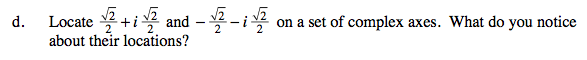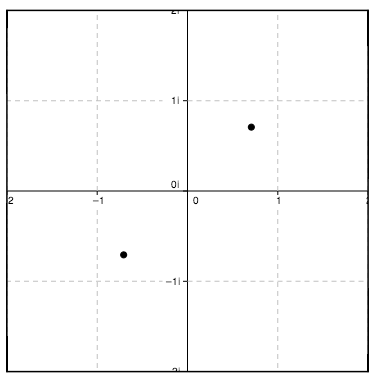Home > A2C > Chapter 11 > Lesson 11.3.3 > Problem11-160

11-160.
1. Compute the value of each expression below. Homework Help ✎

1.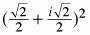2.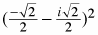3. Use the results from parts (a) and (b) above to solve x2 = i for x. (Find the square roots of i.)

4. Locate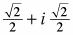and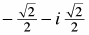on a set of complex axes. What do you notice about their locations?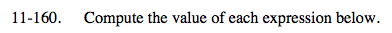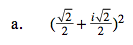$\frac{(2)}{4}+\frac{2i(2)}{4}+\frac{(-1)(2)}{4}$

i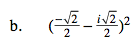i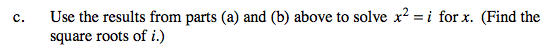Notice that the expressions in parts (a) and (b), when squared, result in the value of i.